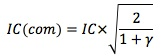### Why should I choose AnalystNotes?

AnalystNotes specializes in helping candidates pass. Period.

### Subject 3. Assumptions

Three main assumptions of the fundamental law of active management:

• The manager can measure his or her own skills (i.e. IC) accurately, and exploits information in an optimal way.

This is the most crucial assumption. Managers need to know what they know, and what they don't know. They need to know how to turn their ideas into portfolios and gain the benefits of their insights.

• The sources of information are independent. This means forecast 2 should not be based on a source of information that is correlated with the sources of forecast 1.

If the information sources are dependent, it then results in an decrease in the overall skill level of a manager.where γ is the correlation between the two information sources.

• The skill involved in forecasting each component, IC, is the same. In other words, the skill curve is horizontal. However, the manager may have greater skills in one area than another.

#### Practice Question 1

Suppose there are two information sources. Each has a level of skill IC. The value of the incremental information is maximized if the correlation between sources is:

A. -1.
B. 0.
C. 1.

It is maximized when there is no correlation.

#### Practice Question 2

If a manager reassesses his industry and marks bets on the basis of new information each year, but rebalances his portfolio monthly, then effectively:

A. He uses 12 information sources.
B. He makes the same bet 12 times.
C. He makes 12 industry bets per year.

#### Practice Question 3

Suppose there are two information sources. The IC for each source is 0.05. The correlation between the two sources is 1. The skill level of the combined sources is:

A. 0.05.
B. 0.055.
C. 0.06.

No calculation is needed as they are perfectly correlated.

#### Practice Question 4

Suppose there are two information sources. The IC for each source is 0.06. The correlation between the two sources is 0.5. The skill level of the combined sources is:

A. 0.065.
B. 0.069.
C. 0.073.

IC(com) = 0.06 (2/1.5)1/2 = 0.069.

#### Practice Question 5

Suppose there are two information sources. The IC for each source is 0.05. The correlation between the two sources is 0. The skill level of the combined sources is:

A. 0.05.
B. 0.06.
C. 0.07.